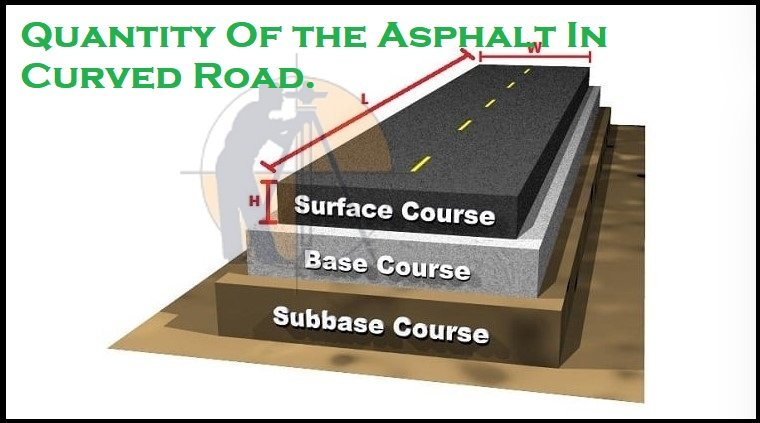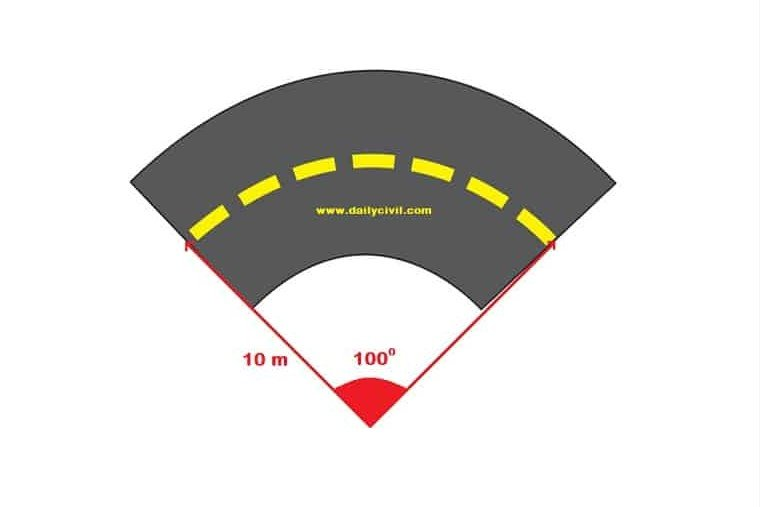Breaking News
Home / Land Surveying / How To Calculate The Asphalt Quantity For Road Work

# How To Calculate The Asphalt Quantity For Road Work

## How To Calculate The Asphalt Quantity For Road.

In this article, I will discuss how to calculate the ( QTY ) Quantity of the Asphalt & its cost for the road construction. The calculation is very easy, u only need some data.

There are many who get confused between the asphalt & bitumen. They think that asphalt & bitumen are the same, but technically they are not same.

Asphalt is the composite material mixture of the aggregates, binder (bitumen) & sand. Aggregates used for the asphalt mix could be crushed rock, sand and gravel, or slags.

Whereas Bitumen is actually the liquid binder that holds the asphalt together. The term bitumen is often mistakenly used to the describe asphalt.

• ### Types Of Scales In Surveying

Asphalt is widely used for the constructing & maintaining all types of the roads be it for highways, inner-city & inter-city roads, local roads and car parks, or paving driveways & pavements. I will briefly discuss the difference between asphalt & bitumen in the another article.### Example,

Length of the road = L = five km = 5000 m

Width of the road = W = Six m

Thickness of the asphalt = H = 15 cm = 0.15 m

1. The Role and Responsibilities of QTY Surveyor in the Current Field Of Construction Sector

### Quantity Of the Asphalt Calculation.

#### The volume of the asphalt = L x W x H

= 5000 x  Six  x 0.15 = 4500 m3

Here, the density of the asphalt is taken as 2330 kg/m3

Therefore, Quantity of the asphalt = Volume of the asphalt x Density of the asphalt

= 4500 x 2330 = 10485000 kg = 10485 tons.

### Cost of the asphalt.

Suppose the cost of One ton asphalt is 6000 rs.

Cost of the asphalt = 10485 x 6000 = 62910000 rs.

### Quantity Of the Asphalt In Curved Road.### Example,

1. Width of the road = W = Six m
2. Thickness of the asphalt = H = 15 cm = 0.15 m

Length of the Curved Road = (π x R x θ)/180 = (3.14 x Ten x 100)/180 = 17.44 m

Volume of the asphalt for curved road = Length x Width x Thickness

= L x W x H = 17.44 x  Six  x 0.15 = 15.696 m3

### Quantity of the asphalt for curved road = Volume of the asphalt x Density of the asphalt.

= 15.69 x 2330 = 36558 kg = 36.55 tons.

### Cost of the asphalt.

Suppose the cost of One ton asphalt is 6000 rs.

Cost of the asphalt = 36.55 x 6000 = 219300 rs

### Note.

In case of the area u are calculating has an irregular shape, divide it into the several regularly-shaped sections, then Calculate each of their volume & asphalt requirements using the above method. Finally sum them the up together.

Visit My YouTube Channel For Land Surveying Basic Knowledge.

## . Land Surveying & Architects

### THANKS.